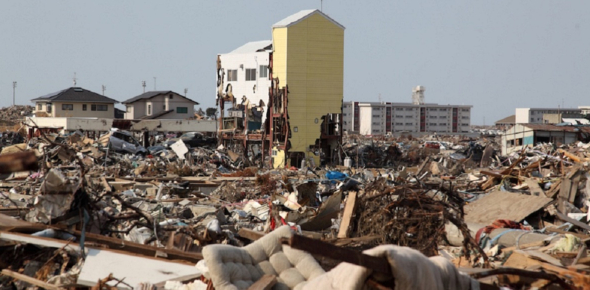# Science: The Ultimate Earthquake Quiz!

16 Questions | Total Attempts: 6719SettingsEarthquakes are formed as a result of tectonic movement in the earth’s crust. The magnitude of an earthquake is directly proportional to how dangerous it is and the damage it will result in. During our science classes we were able to understand how they occur. Just how much did you understand about earthquakes? Take up this test and find out!

• 1.
Once the elastic limit of rocks is passed, they break and move along surfaces called ______.
• A.

Faults

• B.

Earthquakes

• C.

Strains

• D.

Stresses

• 2.
The height of the lines traced on paper is a measure of the energy released of the ________of the earthquake.
• A.

Stress

• B.

Strain

• C.

Magnitude

• D.

Intensity

• 3.
Most earthquakes happen ___________
• A.

Without warning

• B.

In areas where earthquakes have occurred in the past

• C.

Along plate boundaries

• D.

All of the above

• 4.
Scientists discovered changes in Earth's interior by studying _______.
• A.

Tsunamis

• B.

Tides

• C.

Changes in seismic waves

• D.

All of the above

• 5.
____________is the force that squeezes rocks together.
• A.

Tension

• B.

Shear

• C.

Elastic limit

• D.

Compression

• 6.
______________faults are caused by tensional forces.
• A.

Normal

• B.

Strike-slip

• C.

Reverse

• D.

Elastic

• 7.
_____________faults are caused by compressional forces.
• A.

Normal

• B.

Strike-slip

• C.

Reverse

• D.

Elastic

• 8.
The most destructive seismic wave are _____________.
• A.

Primary waves

• B.

Secondary waves

• C.

P-waves

• D.

Surface waves

• 9.
The ____________waves are the first to reach a seismograph after an earthquake.
• A.

Surface

• B.

Secondary

• C.

Primary

• D.

Tsunami

• 10.
At least _____________seismographs are needed to accurately locate an earthquake epicenter.
• A.

Two

• B.

Five

• C.

Four

• D.

Three

• 11.
The point in Earth's interior where the energy release of an earthquake occurs is the ___________.
• A.

Focus

• B.

Epicenter

• C.

Fault

• D.

Inner core

• 12.
The magnitude of an earthquake is measured by the _________________.
• A.

Richter scale

• B.

Moho discontinuity

• C.

Modified Meralli scale

• D.

Elastic limit

• 13.
_____________ is the force that pulls rocks apart.
• A.

Tension

• B.

Shear

• C.

Elastic limit

• D.

Compression

• 14.
_______________is the force that causes plates to move sideways past each other.
• A.

Tension

• B.

Shear

• C.

Elastic limit

• D.

Comprehession

• 15.
_____________faults are caused by shear forces.
• A.

Normal

• B.

Strike-slip

• C.

Reverse

• D.

Elastic

• 16.
All of the following will improve safety factors during an earthquake EXCEPT to __________.
• A.

Study an area's earthquake history

• B.

Stand next to a window

• C.

Make sure hot-water heater are held securely in place

• D.

Take heavy objects down from high shelves

Related TopicsBack to top